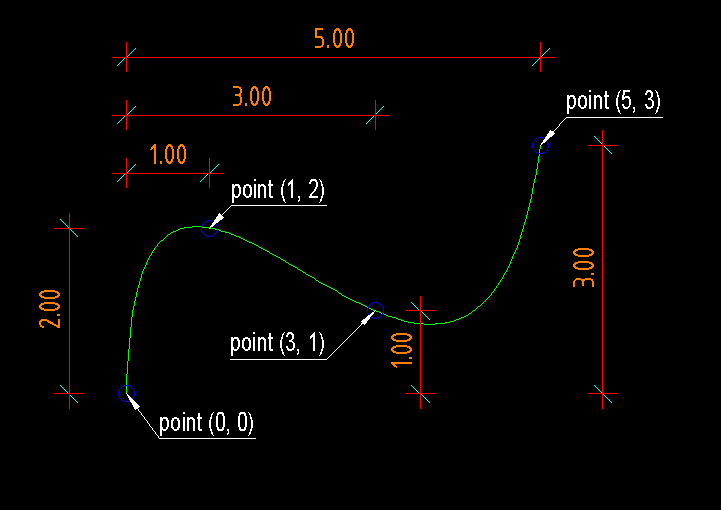# Spline¶

Type: Composite Entity

Spline curves are approximated by POLYLINE.

For an explanation of spline curves see Wikipedia:

http://en.wikipedia.org/wiki/Spline_%28mathematics%29

`DXFEngine.``spline`(points, segments=100, **kwargs)

Create a new cubic-spline-entity, curve shape is an approximation by POLYLINE.

Parameters: points – breakpoints (knots) as 2D points (float-tuples), defines the curve, the curve goes through this points segments (int) – count of line segments for polyline approximation linetype (str) – linetype name, if not defined = BYLAYER layer (str) – layer name color (int) – range [1..255], 0 = BYBLOCK, 256 = BYLAYER

## Example¶

```import dxfwrite
from dxfwrite import DXFEngine as dxf

name = 'spline.dxf'
dwg = dxf.drawing(name)
#spline_points = [(0.0, 0.0), (1., 2.), (3., 1.), (5., 3.)]
spline_points = [(0.0, 0.0), (2., 2.), (3., 2.), (5., 0.)]